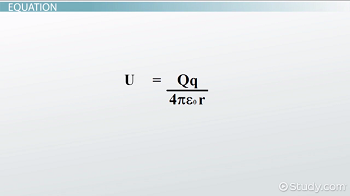# Electric Potential Energy: Definition & Formula

An error occurred trying to load this video.

Try refreshing the page, or contact customer support.

Coming up next: Finding the Electric Potential Difference Between Two Points

### You're on a roll. Keep up the good work!

Replay
Your next lesson will play in 10 seconds
• 0:01 What Is Electric…
• 0:55 Zero of Potential
• 1:27 Equation
• 2:11 Example Calculation
• 3:13 Lesson Summary

Want to watch this again later?

Timeline
Autoplay
Autoplay
Speed

#### Recommended Lessons and Courses for You

Lesson Transcript
Instructor: David Wood

David has taught Honors Physics, AP Physics, IB Physics and general science courses. He has a Masters in Education, and a Bachelors in Physics.

After watching this video, you will be able to explain what electric potential energy is and use an equation to solve electric potential energy problems involving point charges. A short quiz will follow.

## What is Electric Potential Energy?

Electric potential energy is the energy a charge has due to its position relative to other charges. If you take a ball with mass m and raise it to any height, you are giving it gravitational potential energy. We know this for two reasons: one, you have to use energy in your muscles to do it, and two, when you let go of the ball, it falls to the ground and that energy is released again.

Electric potential energy is similar but with charges instead of masses. Instead of raising a ball in the gravitational field of the Earth, you move a charge that's in the electric field of another charge. By separating two charges to a radius r, you are giving the charges electric potential energy relative to each other. When you release those charges, they will attract or repel, releasing that energy. Opposite charges will attract, just like the Earth and the ball. Like charges will repel.

## Zero of Potential

The point where an object has zero potential is an arbitrary value. You can choose it to be wherever you want. This is like how we often measure gravitational potential energy relative to the ground, even though if you moved the ground, a ball would continue to fall until it reached the center of the Earth.

The zero of potential is often put at a distance of zero between two charges for simplicity. But in more advanced physics, for point charges, we tend to put zero at infinity, which means that two charges separated by an infinite distance will have a potential of zero.

## EquationThe equation for electric potential looks like this. Here, U is the electric potential energy between two charges, measured in Joules, big Q is the charge of one of the charges, measured in Coulombs, little q is the charge of the other charge, measured in Coulombs, epsilon-zero is a constant, which is always equal to 8.85 x 10^-12, and r is the distance (or radius) between the charges, measured in meters.

So if you know the sizes of each charge and the distance between them, you can calculate the electric potential energy they have relative to each other. This is assuming the two charges can be treated as point charges, which are where all the charge is concentrated at an exact point in space. Extended objects get more complex and require some calculus.

## Example Calculation

Okay, let's go through an example. Let's say you have two particles: one is an electron, and the other is some unknown particle that has a charge of 8 * 10^-19 Coulombs. If the two particles are 2 * 10^-11 meters apart, how much electric potential energy do they have relative to each other?

To unlock this lesson you must be a Study.com Member.

### Register to view this lesson

Are you a student or a teacher?

### Unlock Your Education

#### See for yourself why 30 million people use Study.com

##### Become a Study.com member and start learning now.
Back
What teachers are saying about Study.com

### Earning College Credit

Did you know… We have over 160 college courses that prepare you to earn credit by exam that is accepted by over 1,500 colleges and universities. You can test out of the first two years of college and save thousands off your degree. Anyone can earn credit-by-exam regardless of age or education level.